# Finding the area of a right triangle on a grid Online Quiz

Following quiz provides Multiple Choice Questions (MCQs) related to Finding the area of a right triangle on a grid. You will have to read all the given answers and click over the correct answer. If you are not sure about the answer then you can check the answer using Show Answer button. You can use Next Quiz button to check new set of questions in the quiz.Q 1 - Find the area of the given right triangle on a grid.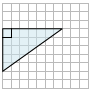### Explanation

Step 1:

Area of Triangle = $\frac{1}{2}$ × b × h; b = base = 7; h = height = 5

Step 2:

Area of given right triangle on a grid = $\frac{1}{2}$ × 7 × 5 = 17.5 square units

Q 2 - Find the area of the given right triangle on a grid.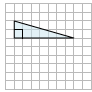### Explanation

Step 1:

Area of Triangle = $\frac{1}{2}$ × b × h; b = base = 7; h = height = 2

Step 2:

Area of given right triangle on a grid = $\frac{1}{2}$ × 7 × 2 = 7 square units

Q 3 - Find the area of the given right triangle on a grid.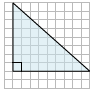### Explanation

Step 1:

Area of Triangle = $\frac{1}{2}$ × b × h; b = base = 9; h = height = 8

Step 2:

Area of given right triangle on a grid = $\frac{1}{2}$ × 9 × 8 = 36 square units

Q 4 - Find the area of the given right triangle on a grid.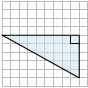### Explanation

Step 1:

Area of Triangle = $\frac{1}{2}$ × b × h; b = base = 9; h = height = 5

Step 2:

Area of given right triangle on a grid = $\frac{1}{2}$ × 9 × 5 = 22.5 square units

Q 5 - Find the area of the given right triangle on a grid.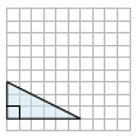### Explanation

Step 1:

Area of Triangle = $\frac{1}{2}$ × b × h; b = base = 6; h = height = 3

Step 2:

Area of given right triangle on a grid = $\frac{1}{2}$ × 6 × 3 = 9 square units

Q 6 - Find the area of the given right triangle on a grid.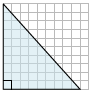### Explanation

Step 1:

Area of Triangle = $\frac{1}{2}$ × b × h; b = base = 9; h = height = 10

Step 2:

Area of given right triangle on a grid = $\frac{1}{2}$ × 9 × 10 = 45 square units

Q 7 - Find the area of the given right triangle on a grid.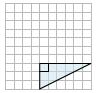### Explanation

Step 1:

Area of Triangle = $\frac{1}{2}$ × b × h; b = base = 6; h = height = 3

Step 2:

Area of given right triangle on a grid = $\frac{1}{2}$ × 6 × 3 = 9 square units

Q 8 - Find the area of the given right triangle on a grid.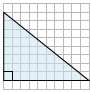### Explanation

Step 1:

Area of Triangle = $\frac{1}{2}$ × b × h; b = base = 10; h = height = 8

Step 2:

Area of given right triangle on a grid = $\frac{1}{2}$ × 10 × 8 = 40 square units

Q 9 - Find the area of the given right triangle on a grid.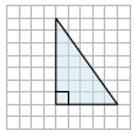### Explanation

Step 1:

Area of Triangle = $\frac{1}{2}$ × b × h; b = base = 5; h = height = 7

Step 2:

Area of given right triangle on a grid = $\frac{1}{2}$ × 5 × 7 = 17.5 square units

Q 10 - Find the area of the given right triangle on a grid.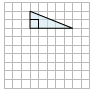Area of Triangle = $\frac{1}{2}$ × b × h; b = base = 5; h = height = 2
Area of given right triangle on a grid = $\frac{1}{2}$ × 5 × 2 = 5 square units